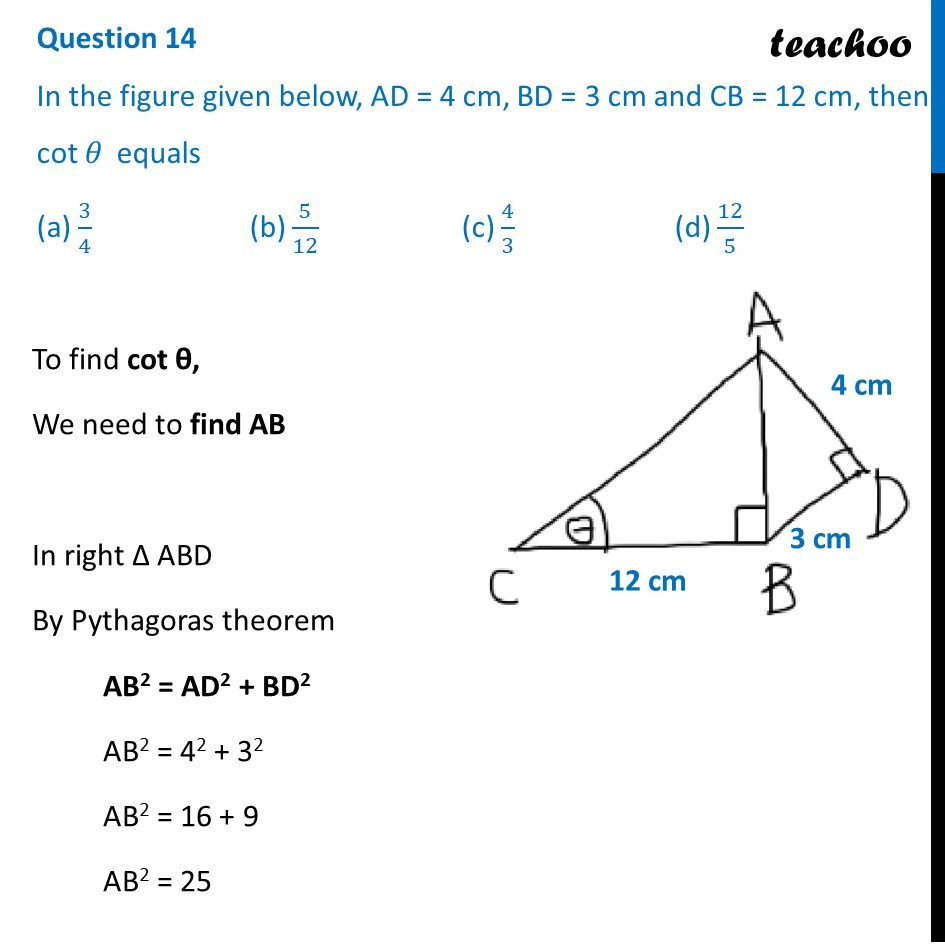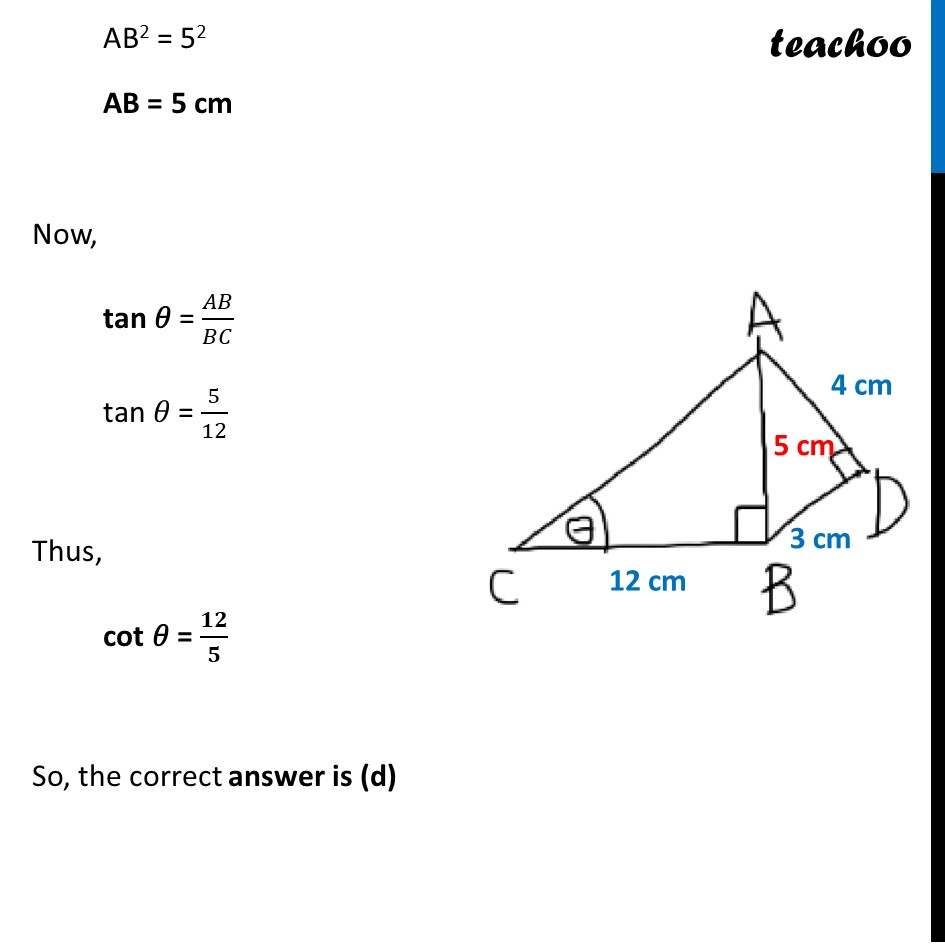## (a) 3/4  (b) 5/12   (c) 4/3   (d) 12/51. Class 10
2. Solutions of Sample Papers for Class 10 Boards
3. CBSE Class 10 Sample Paper for 2022 Boards - Maths Basic [MCQ]

Transcript

Question 14 In the figure given below, AD = 4 cm, BD = 3 cm and CB = 12 cm, then cot 𝜃 equals (a) 3/4 (b) 5/12 (c) 4/3 (d) 12/5 To find cot θ, We need to find AB In right Δ ABD By Pythagoras theorem AB2 = AD2 + BD2 AB2 = 42 + 32 AB2 = 16 + 9 AB2 = 25 AB2 = 52 AB = 5 cm Now, tan 𝜃 = 𝐴𝐵/𝐵𝐶 tan 𝜃 = 5/12 Thus, cot 𝜃 = 𝟏𝟐/𝟓 So, the correct answer is (d)